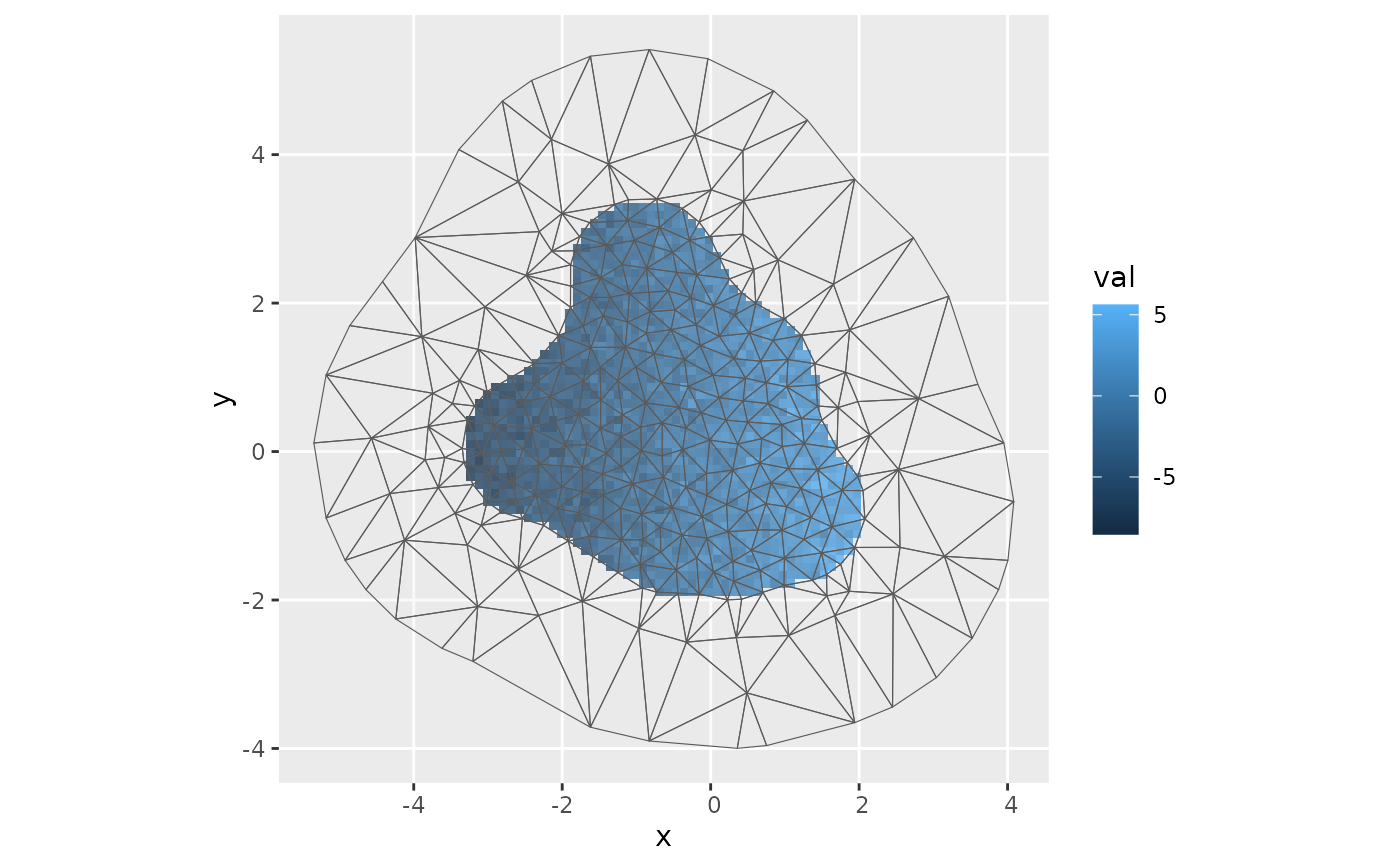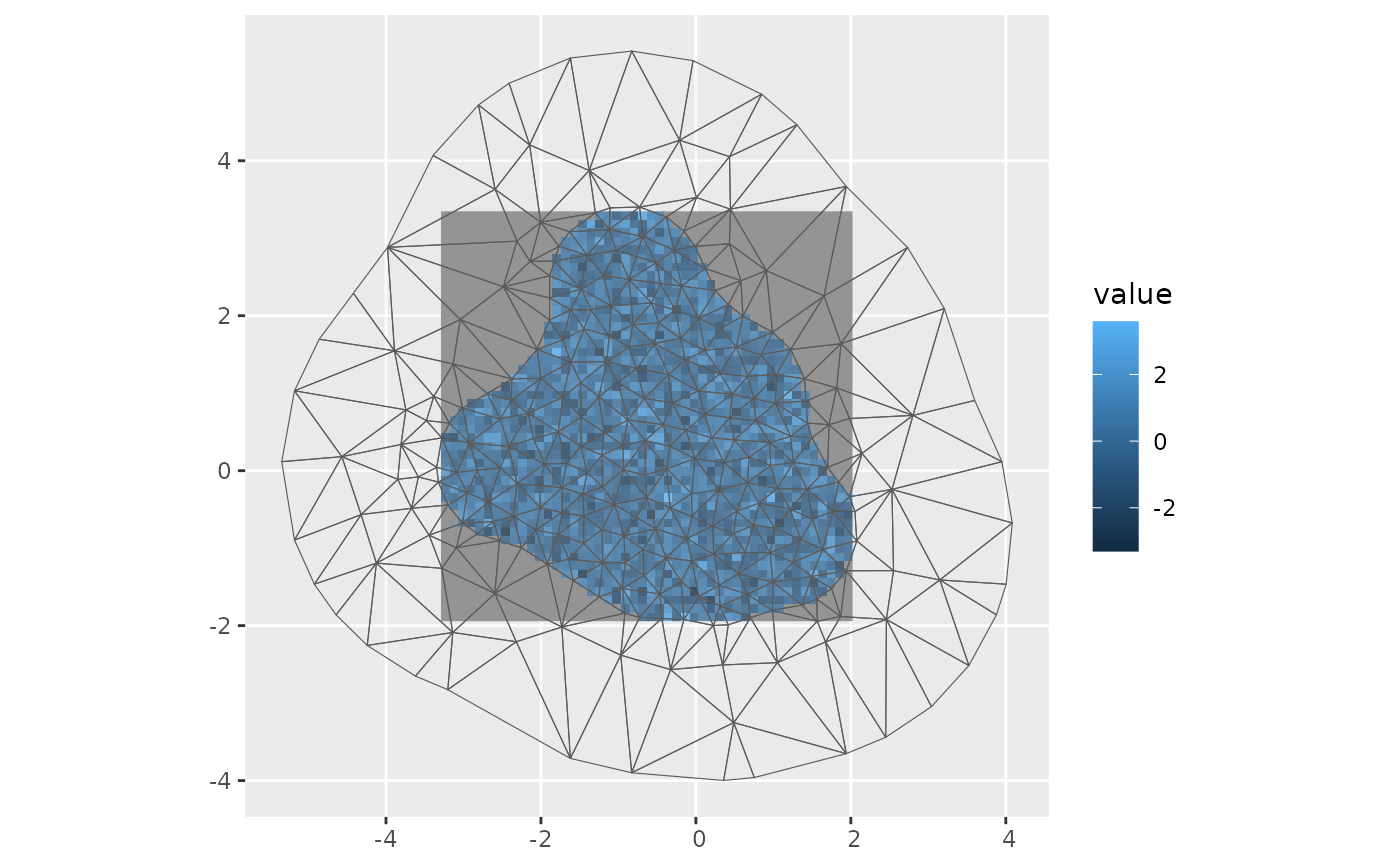Generate terra, sf, or sp lattice locations

## Usage

fm_pixels(
mesh,
dims = c(150, 150),
xlim = NULL,
ylim = NULL,
format = "sf",
minimal = TRUE,
nx = deprecated(),
ny = deprecated()
)

## Arguments

mesh

An fm_mesh_2d object

dims

A length 2 integer vector giving the dimensions of the target lattice.

xlim, ylim

Length 2 numeric vectors of x- and y- axis limits. Defaults taken from the range of the mesh or mask; see minimal.

If logical and TRUE, remove pixels that are outside the mesh. If mask is an sf or Spatial object, only return pixels covered by this object.

format

character; "sf", "terra" or "sp"

minimal

logical; if TRUE (default), the default range is determined by the minimum of the ranges of the mesh and mask, otherwise only the mesh.

nxNumber of pixels in x direction, or a numeric vector of x-values

nyNumber of pixels in y direction, or a numeric vector of y-values

## Value

sf, SpatRaster, or SpatialPixelsDataFrame covering the mesh or mask.

## Author

Finn Lindgren finn.lindgren@gmail.com

## Examples

if (require("ggplot2", quietly = TRUE)) {
dims <- c(50, 50)
pxl <- fm_pixels(
fmexample$mesh, dims = dims, mask = fmexample$boundary_sf[],
minimal = TRUE
)
pxl$val <- rnorm(NROW(pxl)) + fm_evaluate(fmexample$mesh, pxl, field = 2 * fmexample$mesh$loc[, 1])
ggplot() +
geom_tile(
data = pxl,
aes(geometry = geometry, fill = val),
stat = "sf_coordinates"
) +
geom_sf(data = fm_as_sfc(fmexample$mesh), alpha = 0.2) }# \donttest{ if (require("ggplot2", quietly = TRUE) && require("terra", quietly = TRUE) && require("tidyterra", quietly = TRUE)) { pxl <- fm_pixels(fmexample$mesh,
dims = c(50, 50), mask = fmexample$boundary_sf[], format = "terra" ) pxl$val <- rnorm(NROW(pxl) * NCOL(pxl))
pxl <-
pxl,
mask = pxl$.mask, maskvalues = c(FALSE, NA), updatevalue = NA ) ggplot() + geom_spatraster(data = pxl, aes(fill = val)) + geom_sf(data = fm_as_sfc(fmexample$mesh), alpha = 0.2)
}
#> terra 1.7.55
#>
#> Attaching package: ‘terra’
#> The following objects are masked from ‘package:splancs’:
#>
#>     as.points, is.points, zoom
#>
#> Attaching package: ‘tidyterra’
#> The following object is masked from ‘package:stats’:
#>
#>     filter# }